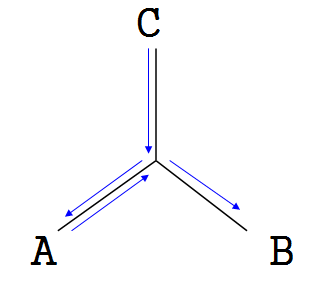# 洛谷 P4408 [NOI2003]逃学的小孩 题解【树的直径】【贪心】

## 题目描述

Chris家的电话铃响起了，里面传出了Chris的老师焦急的声音：“喂，是Chris的家长吗？你们的孩子又没来上课，不想参加考试了吗？”一听说要考试，Chris的父母就心急如焚，他们决定在尽量短的时间内找到Chris。他们告诉Chris的老师：“根据以往的经验，Chris现在必然躲在朋友Shermie或Yashiro家里偷玩《拳皇》游戏。现在，我们就从家出发去找Chris，一但找到，我们立刻给您打电话。”说完砰的一声把电话挂了。

Chris居住的城市由N个居住点和若干条连接居住点的双向街道组成，经过街道x需花费Tx分钟。可以保证，任两个居住点间有且仅有一条通路。Chris家在点C，Shermie和Yashiro分别住在点A和点B。Chris的老师和Chris的父母都有城市地图，但Chris的父母知道点A、B、C的具体位置而Chris的老师不知。

1. 如果A距离C比B距离C近，那么Chris的父母先去Shermie家寻找Chris，如果找不到，Chris的父母再去Yashiro家；反之亦然。
2. Chris的父母总沿着两点间唯一的通路行走。

## 输入输出样例

4 3
1 2 1
2 3 1
3 4 1

4
题目中所给的A，B，C三点是不确定的，但是“两点间有唯一的通路”，也就是说，给出一棵树，题目要求出C点到A，B点中较近的一个，然后加上A到B的距离。我们如果用d(i,j)表示i到j的距离，那么答案就是对于所有不同的点A，B，C，要求最大的$min(d(C,A),d(C,B))+d(A,B)$。在树上，我们可以知道，假定$d(C,A)<d(C,B)$ ，如果B是A的儿子，那么家长肯定先到B再到A；如果B和A有公共祖先且不是A或B（即A，B不属于同一棵子树），那么他们的路径一定是这样的；可见，在最坏一种情况中，总会有一段路径在直径上，即A和B分属直径两端。【贪心】
我们联想到树网的核中偏心距的概念，就是直径外一点到直径的最短距离。然而从图中我们可以发现，C到中间的点（称之为D）的距离是偏心距，此时，$|DA|<|DB|$ ，所以要加上DA【贪心*2】【树的直径】
因此我们需要两步：一、求树的直径；二、求直径上的点能到达的最远距离，并加上该点到直径端点的较近距离。
树的直径求法：我们从任意一个点出发做DFS，找到的距离最远的点就是直径的一个端点（这里不给出证明），再从端点出发，找到最远的点是直径的另一端点。我们可以在回溯过程中将直径找出来，也可以重新做DFS。
偏心距求法：在树的直径上枚举点，因为任意两点之间的路是唯一的，如果直径上一点能到达T点，直径上就不会有另一点能到达T点了。所以从每点出发做一次DFS，就能在$O(N)$的时间内把这个工作处理出来。
最后再枚举所有点的偏心距加上到最近端点的和的最大值，加上直径长度，就能出答案了。

## Code：

#include<cstdio>
#include<cstring>
#include<algorithm>
using std::max;
using std::min;
const int N=200800;
struct node
{
int n;
long long v;
node *nxt;
node(int n,long long v)
{
this->n=n;
this->v=v;
nxt=NULL;
}
node(){nxt=NULL;}
};
long long far=0,maxx=0;
int D,t;//D是直径的端点
bool used[N];
void dfs(int x)
{
if(far>maxx)
{
maxx=far;
D[t]=x;
}
while(p->nxt!=NULL)
{
p=p->nxt;
if(used[p->n])
continue;
far+=p->v;
used[p->n]=true;
dfs(p->n);
used[p->n]=false;
far-=p->v;
}
}
long long d[N],s[N];
int cnt=0,flag;
//d是存直径上的点，s[i]存d[i]到d[i+1]的边长
void Dfs(int x)
{
if(x==D)
{
d[++cnt]=x;
flag=1;
return;
}
while(p->nxt!=NULL)
{
p=p->nxt;
if(used[p->n])
continue;
used[p->n]=true;
Dfs(p->n);
used[p->n]=false;
if(flag==1)
{
s[cnt]=p->v;
d[++cnt]=x;
break;
}
}
}
long long l[N],sum=0;//这是到直径的距离。
{
while(p->nxt!=NULL)
{
p=p->nxt;
if(used[p->n])
continue;
used[p->n]=true;
sum+=p->v;
sum-=p->v;
used[p->n]=false;
}
}
long long b[N];
int main()
{
memset(b,0,sizeof(b));
memset(used,0,sizeof(used));
int n,m,u,v;
long long w;
scanf("%d%d",&n,&m);
for(int i=1;i<=n;i++)
for(int i=1;i<=m;i++)
{
scanf("%d%d%lld",&u,&v,&w);
tail[u]->nxt=new node(v,w);
tail[u]=tail[u]->nxt;
tail[v]->nxt=new node(u,w);
tail[v]=tail[v]->nxt;
}
t=1;
used=true;
dfs(1);//O(N)
t=2;
maxx--;
memset(used,0,sizeof(used));
used[D]=true;
dfs(D);//O(N)
memset(used,0,sizeof(used));
flag=0;
used[D]=true;
Dfs(D);//找d2，回溯直径上的点
//做前缀和b[i]指i到D的距离（D是d）
for(int i=2;i<=cnt;i++)//直径的长度直接用b[cnt]表示
b[i]=b[i-1]+s[i-1];
//让直径上每一个点去找这一点的偏心距
memset(used,0,sizeof(used));
for(int i=1;i<=cnt;i++)
used[d[i]]=1;
for(int i=1;i<=cnt;i++)
{
sum=0;//不用处理直径上的点，可以直接带进去处理
DFs(d[i],min(b[i],b[cnt]-b[i]));
}
long long ans=0;
for(int i=1;i<=n;i++)
if(l[i]>ans)
ans=l[i];
/*for(int i=1;i<=n;i++)
printf("%d ",l[i]);*/
printf("%lld\n",ans+b[cnt]);
return 0;
}


### 1 说点什么0 Followers

Most reacted comment
0 Comment authors
Recent comment authors
SubscribewpDiscuz